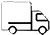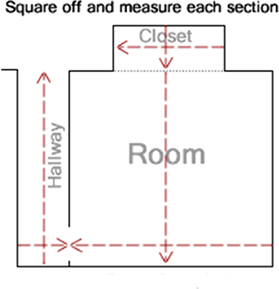Shipping Nationwide. Call (844) 999-2626

# How to Measure Your Room

Hardwood flooring is sold by the square foot so take the length of the room and multiply it by the width. For regions with edges, measure to the longest points and divide that number by 2. This will give you the aggregate square feet of the room.

Here are a couple of examples below:

Room Dimension for each sample are:

• 10 feet long by 9 feet wide, which multiplied is 90 sq ft (10 x 9 = 90).
• 13 feet 6 inches long by 15 feet 4 inches wide. It’s best to round both numbers to the next foot. So, you would go with 14 feet (long) x 16 feet (wide) which multiplied is 224 sq ft (14 x 16 = 224).

Some prefer to measure more accurately by measuring by the inch. Measure the length and width of the room in inches and multiply those numbers.

Here’s an example of measuring by inches:

Room Dimension in inches listed below:

• 348 inches long by 274 inches wide, multiply those numbers and it comes to 94,352 inches. Divide that by the number of square inches in a square foot, which is 144, and you have 662.17 square feet.

Industry standard suggest adding 8% to 10% waste for corners, cut backs, walls, etc. for higher quality products. Take the amount of sq ft and 8% to 10%. Ordering a more Rustic or Utility Grade of hardwood flooring will come with a higher waste factor that may include anywhere from 12% up to 30%. Add up all the rooms you are installing hardwood flooring into and you come up with the amount of square footage needed for your project.• Square off each section of the area you are going to install hardwood flooring so you can measure the width and length to calculate the square footages.
• Then add the square footage for each section together to get the total square footage for the area you plan on installing flooring in.
• Add 10% to the total square footage for waste and extra pieces. (Width * Length = Total Square Feet * 1.10)

Note: Most hardwood flooring is sold in full cartons only. So you will need to know how many square feet are in a carton. Then divide your total square footage by the square feet per carton and round up to the nearest carton.

## Compare products

{"one"=>"Select 2 or 3 items to compare", "other"=>"{{ count }} of 3 items selected"}

Select first item to compare

Select second item to compare

Select third item to compare